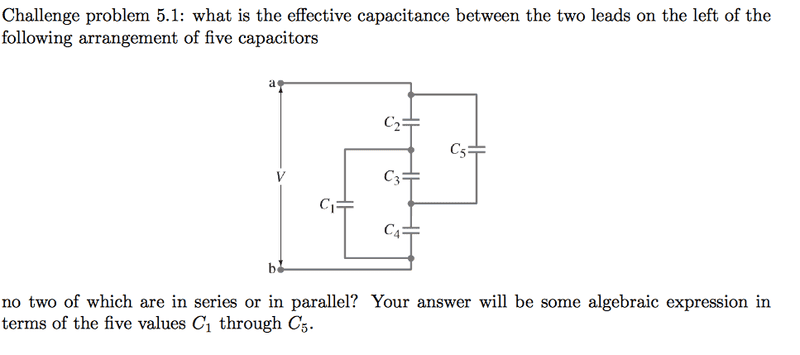# Difficult Introductory Electricity Circuit Problem - Capacitors

Difficult Introductory Electricity Circuit Problem -- Capacitors

## Homework Statement## The Attempt at a Solution

This problem was very difficult, and the most I could do was re-arrange the circuit into a make-shift Wheatstone bridge with all capacitors instead of resistors/voltmeter.

Any ideas?

Last edited:

## Answers and Replies

BvU
Science Advisor
Homework Helper
What you need is a relevant equation ! Hence the template...

I propose Q = C V There are five of them, plus one for the equivalent over-all C, so you should get enough material to solve for the latter.

That's how we do parallel, series, etc. of two capacitors. This one is a little more involved.

I've already attempted doing that - I think the big problem is that since no two of them are in series/parallel with each other, it's very difficult to use the standard method.

BvU
Science Advisor
Homework Helper
Show what you did, it might be just right, only a bit confusing

I scrapped my approach because it wasn't working.

Do you mean I should calculate the charge on the capacitors using Q = VC?

SammyS
Staff Emeritus
Science Advisor
Homework Helper
Gold Member

## Homework Statement

[ IMG]http://i.imgur.com/MAvH81u.png[/PLAIN]

## The Attempt at a Solution

This problem was very difficult, and the most I could do was re-arrange the circuit into a make-shift Wheatstone bridge with all capacitors instead of resistors/voltmeter.

Any ideas?
Are the capacitances all different, or do all capacitors have the same capacitance?

#### Attachments

They're all different...does that make a difference? I figure the calculation would be the same.

NascentOxygen
Staff Emeritus
Science Advisor
They're all different...does that make a difference? I figure the calculation would be the same.
The special case of all being equal allows you to make a drastic simplification right from the start, basing this on symmetry of the circuit. For this reason, the special case cannot qualify as a "challenge problem".•1 person
berkeman
Mentor

## Homework Statement## The Attempt at a Solution

This problem was very difficult, and the most I could do was re-arrange the circuit into a make-shift Wheatstone bridge with all capacitors instead of resistors/voltmeter.

Any ideas?

I just write the KCL equations for complicated questions like this.